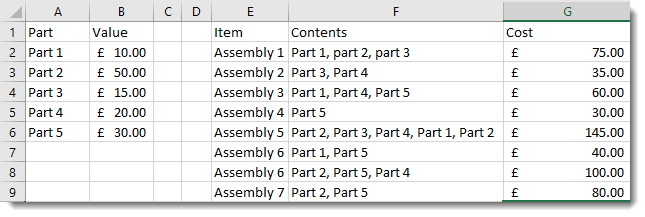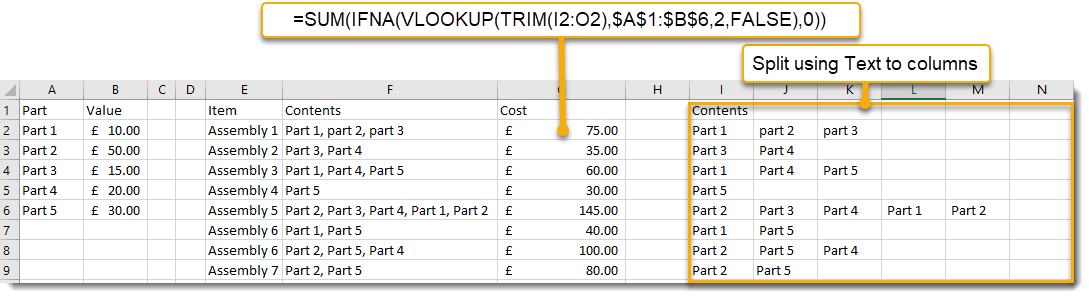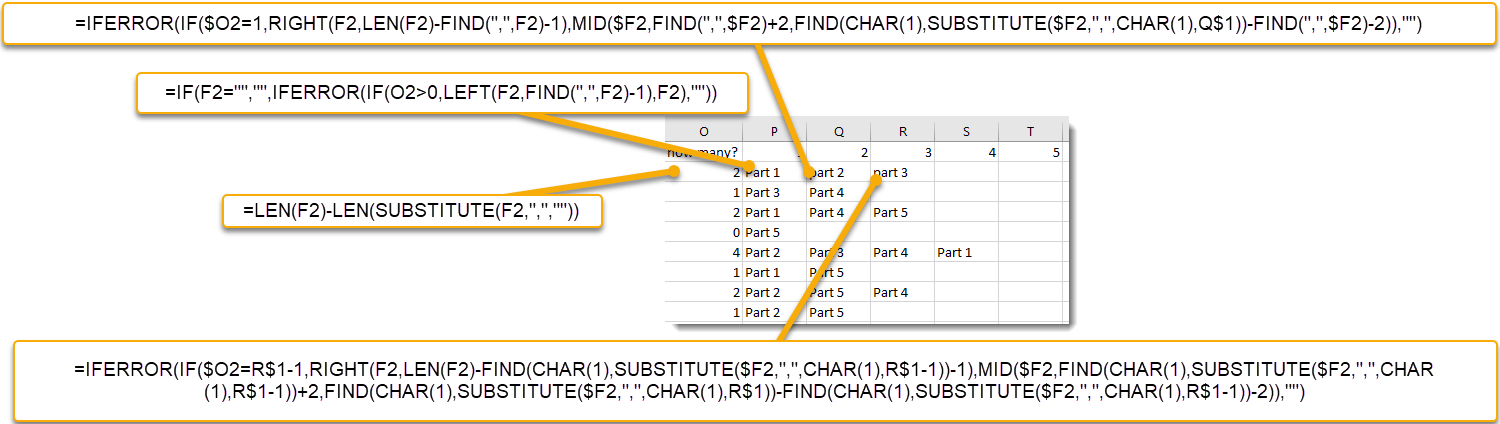Home > Blog

# Sum VLOOKUP across a whole table - a fast way to do many Vlookup calculations

If you know about VLOOKUP or XLOOKUP you will know that it is a very powerful way to pull data in from one list to be used in calculations or displayed.  This blog will demonstrate a quick way to use it against many items.

In the example below we have a list of parts and need to total up how much they will cost.  A VLOOKUP is a natural way to identify that Part 1 costs £10.00 and Parts 2 and 3 are £50 and £15 respectively so assembly 1 will cost £75.00.  Normally when doing this we would use three VLOOKUP expressions to pull the data from the table in columns A and B and then add up the results.However, there is a faster way.G2 =SUM(IFNA(VLOOKUP(TRIM(I2:O2),\$A\$1:\$B\$6,2,FALSE),0))

This expression allows each of the items in I2:02 to be used in a VLOOKUP and all of these results are then added together using the SUM function.

The TRIM removes any extraneous spaces before or after each of the entries in I:O.

The IFNA is used to include a 0 if the VLOOKUP results in an error.

Splitting the Contents out to allow the VLOOKUP to work can be done in two ways.

The simplest is using the Text to Columns function in the Data menuThis allows you to quickly split the Contents cells apart using the "," as a separator.   This will need to be repeated whenever the Contents column changes.

A much more complex method uses Left, Mid and Right to split the cells apart:The formulas are:

O2 =LEN(F2)-LEN(SUBSTITUTE(F2,",",""))

P2 =IF(F2="","",IFERROR(IF(O2>0,LEFT(F2,FIND(",",F2)-1),F2),""))

Q2 =IFERROR(IF(\$O2=1,RIGHT(F2,LEN(F2)-FIND(",",F2)-1),MID(\$F2,FIND(",",\$F2)+2,FIND(CHAR(1),SUBSTITUTE(\$F2,",",CHAR(1),Q\$1))-FIND(",",\$F2)-2)),"")

R2 =IFERROR(IF(\$O2=R\$1-1,RIGHT(F2,LEN(F2)-FIND(CHAR(1),SUBSTITUTE(\$F2,",",CHAR(1),R\$1-1))-1),MID(\$F2,FIND(CHAR(1),SUBSTITUTE(\$F2,",",CHAR(1),R\$1-1))+2,FIND(CHAR(1),SUBSTITUTE(\$F2,",",CHAR(1),R\$1))-FIND(CHAR(1),SUBSTITUTE(\$F2,",",CHAR(1),R\$1-1))-2)),"")

R2 is copied as many times as necessary to the right

A short explanation of what these formulas are doing:

O2 is counting how many commas there are in the contents cell (f2) by substituting "" for "," and measuring the difference in length.

P2 is checking the F2 has anything in, if it does it tries to return the left-most element of the contents cell, however, if this fails (because there isn't a comma) it returns the whole of F2.

Q2 either returns the right-most element or it takes finds the 2nd element buy using the formula FIND(CHAR(1),SUBSTITUTE(\$F2,",",CHAR(1),Q\$1)) to find the nth comma - driven by the contents of Q2

R2 and the items to the right either return the right-most string element or uses the Nth technique to return a Mid value driven by R1.Miles Goodchild has been a Program and Programme Planner, PMO lead and Project Manger since 1998.  He enjoys using MS Office, especially Project, Excel and Visio to make life easier and simpler.  In the course of this he created SummaryPro.  In this blog he shares some of the tips and techniques he has learnt over the years in the hope that they will be useful to you.